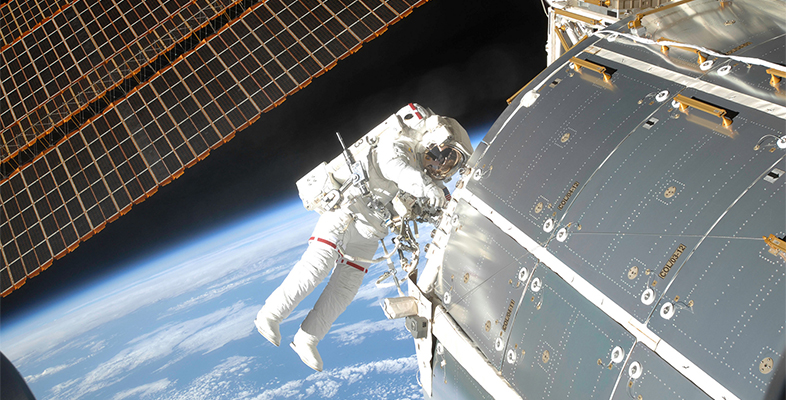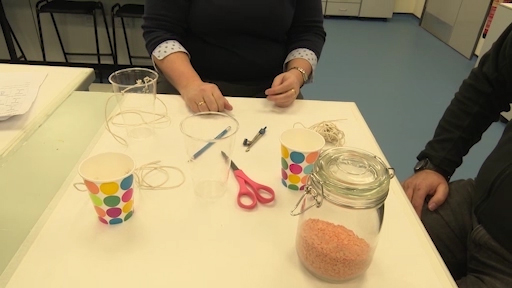Microgravity: living on the International Space Station

Start this free course now. Just create an account and sign in. Enrol and complete the course for a free statement of participation or digital badge if available.

Free course

# 4.1 Practical experiment 1Skip transcript: Video 5 Demonstration of Practical Experiment.

#### Transcript: Video 5 Demonstration of Practical Experiment.

HELEN:
So Tom and I are now back inside in the warm in the lab. And we've gathered together all the things we need to do the experiment about motion in a circle. First of all, we've got a jar of lentils, pasta, rice. Anything like that will do. Second, we've got some plastic cups. This one is a clear one. This one is a paper one. Either one is just as good. And what we need to do is we need to take the cup, and we need to make a small hole on either side of the cup.
Now, I happen to be using the point of a compass, but you could use scissors or actually a pencil. Either one will do. And what you need to do is thread some string through each side of the cup to make something like this, which Tom made earlier-- a piece of string with two knots on it that can hang equally from either side of the cup. And now what I'm going to do is I'm going to ask Tom to fill the cup as bravely as he feels like with some lentils from the jar. Can you do that, Tom?
TOM:
Thank you, Helen. Well, I'm going to take the jar of lentils. I'm going to place some of these into the cup. I think that should be enough.
HELEN:
OK, Tom. Why don't you go and give that cup a whirl around in a circle?
TOM:
I shall certainly try, Helen. Thank you.
HELEN:
Now, the important thing for Tom to do is not to spill any of the lentils. Let's see if it's possible.
[LAUGHTER]
Well done, Tom.
TOM:
Thank you, Helen.
[CLAPPING]
There we go, and not a single lentil lost.
HELEN:
Wow, Tom. That was amazing. But I'm really confused. At some points, the cup was clearly upside down. How did the lentils not all just fall out?
TOM:
And it's amazing when you look at that. The fact that the lentils don't fall out, the reason behind that is the forces are balanced. So as it's going round in circular motion, the forces are balanced so that the lentils stay in as it's going round in a circle, very similar to what happens in the International Space Station. Now, you need to look at the text now as we move again to talking about circular motion and the International Space Station, how it stays in orbit around the Earth.
End transcript: Video 5 Demonstration of Practical Experiment.
Video 5 Demonstration of Practical Experiment.
Interactive feature not available in single page view (see it in standard view).

Practical experiment 1: Modelling how the ISS stays in motion around the Earth with the cup-of-rice experiment

To do this experiment, you need to be in a place with lots of space, ideally in a garden or a field. You will need a plastic cup, a piece of string and some rice. Cut two holes in the plastic cup opposite each other, thread the string through and tie it off. Half-fill the cup with rice. Taking care to avoid hitting anyone, swing this briskly around in a vertical circle.

To keep the cup moving, you need to exert a force (in the string, called tension). To increase the force on the cup, you need to increase the speed. If you put more rice into the cup, the mass has increased, so it will move slower. These are all factors which will change the motion of the cup. These variables are included in Equation 3 where F = force (N), m = mass (kg), v = velocity (speed) and r = radius (m).

Equation label: Equation 3

If the mass m increases, the force F increases (when r and v are constant).

If v increases, F increases (when r and m are constant).

However, if r increases, Fdecreases (when v and m are constant).

If m = 5 kg, and you swing it on a string where r = 1 m and v = 4 m/s, then, using Equation 3, you have a resultant force with a numerical value, as shown in Equation 4.

Equation label: Equation 4

Note that, while the forces are balanced in the rice cup experiment, the rice itself is in freefall while in motion, so that it doesn’t fall out of the cup. You know from Equation 3 that the units for force in Equation 4 should be N. This is the same as kg m/s2.

In terms of the orbit of the ISS, obviously there is no string connecting it to Earth! There must be some other kind of force acting on it. The gravitational pull of Earth is the force F acting here. However, if you then add the effects of friction from the atmosphere, the ISS slows down. It is no longer able to maintain the same gravitational orbit and you would have to either increase v or push it out to a higher orbit.

MG_1